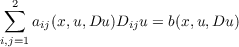#### Vol. 69, No. 1, 1977

 Download this articleFor screen For printingRecent Issues Vol. 325: 1  2 Vol. 324: 1  2 Vol. 323: 1  2 Vol. 322: 1  2 Vol. 321: 1  2 Vol. 320: 1  2 Vol. 319: 1  2 Vol. 318: 1  2Online Archive Volume: Issue:The Journal Subscriptions Editorial Board Officers Contacts Submission Guidelines Submission Form Policies for Authors ISSN: 1945-5844 (e-only) ISSN: 0030-8730 (print) Special Issues Author Index To Appear Other MSP Journals
Equations of mean curvature type in 2 independent variables

### Leon M. Simon

Vol. 69 (1977), No. 1, 245–268
##### Abstract

The object of this paper is to develop a regularity theory for equations of mean curvature type in two independent variables. An equation of mean curvature type in two independent variables is defined to be an equation of the formon a domain Ω R2, where the functions aij, b satisfy special structural conditions. Namely, we require that (i) (1 + |Du|2)12b(x,u,Du) is bounded by a fixed constant (independent of u), and (ii) the quadratic form i,j=12aij(x,u,Du)ξiξj is bounded from above and below in terms of the quadratic form i,j=12gij(Du)ξiξj, where gij(Du) = δij DiuDju∕(1 + |Du|2), i,j = 1,2, are the coefficients of the minimal surface equation.

##### Mathematical Subject Classification 2000
Primary: 49F10, 49F10
Secondary: 35J20
##### Milestones